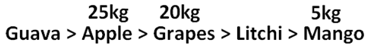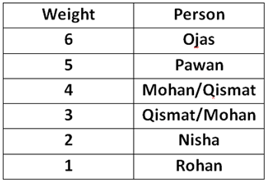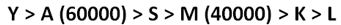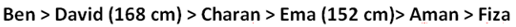Latest Banking jobs   »

# Reasoning Ability Quiz For Bank Foundation 2023- 17th January

Directions (1-3): Study the following information carefully and answer the below questions.
Rama bought five fruits with different weights. The weight of apple is more than the weight of Grapes. Rama bought 5kg of Mango which is the least weight among other fruits. Weight of Litchi is less than the weight of apple which is not the heaviest. She bought 25kg of apple. Weight of Guava is more than Grapes which is not second lowest weight.

Q1. How many fruits are more weights than Litchi?
(a) 2
(b) 3
(c) 1
(d) 4
(e) None

Q2. If the sum of the weights of Grapes and Mango is 25kg, then what is the sum of the weights of Grapes and apples?
(a) 50kg
(b) 40kg
(c) 45kg
(d) 35kg
(e) None

Q3. As many fruits weight is more than Guava is same as less than____?
(a) Litchi
(b) Mango
(c) Apple
(d) Grapes
(e) None of the above

Direction (4-6): Study the following information carefully and answer the questions below.
There are six persons Mohan, Nisha, Ojas, Pawan, Qismat and Rohan of different weights. Nisha is heavier than only Rohan. Two persons are either lighter than Qismat or heavier than Qismat. Pawan is heavier than Mohan but not the heaviest one.

Q4. If Mohan is heavier than only two persons than what is the position of Qismat?
(a) 2nd heaviest person
(b) Heavier than Mohan
(c) 3rd lighter person
(d) Lighter than Mohan
(e) None of these

Q5. Who among the following is heaviest?
(a) Qismat
(b) Mohan
(c) Nisha
(d) Pawan
(e) Ojas

Q6. If the weight of Nisha is 45kg then what may be the weight of Rohan?
(a) 60 Kg
(b) 48 Kg
(c) 56 Kg
(d) 90 Kg
(e) None of these

Directions (7-9): Study the following information carefully and answer the given questions:
Six persons with different monthly salaries are arranged in descending order as per their earnings. S earns more than K but less than A, who does not earn the highest salary. M earns less than Y but more than L who doesn’t earn more than K. S earns more than M. M does not earn the 2nd lowest salary. The second highest and third-lowest salaried persons earn RS. 60000 and 40000 respectively.

Q7. Who among the following person earns the highest salary?
(a) Y
(b) M
(c) L
(d) A
(e) None of these

Q8. If the difference between the salaries of L and A is Rs. 30000, then what will be the sum of monthly salaries of M and L?
(a) 59000
(b) 70000
(c) 57000
(d) 52000
(e) None of these

Q9. Who among the following person earns just less than A?
(a) K
(b) M
(c) S
(d) L
(e) None of these

Directions (10-12): Study the following information carefully and answer the below questions.
Six persons Aman, Ben, Charan, David, Ema and Fiza are comparing their heights and all the persons are at different heights. More than two persons are taller than Ema. David is only shorter than Ben. Both Aman and Fiza are shorter than Charan. Neither Aman nor Fiza taller than Ema. Aman is not shortest among all. The height of the second tallest person is 168 cm and the fourth tallest person is 152 cm.

Q10. What is the possible height of Charan?
(a) 169 cm
(b) 170 cm
(c) 160 cm
(d) 150 cm
(e) 180 cm

Q11. If the lowest height is 7 cm less than Ema’s height, then what is the height of Fiza?
(a) 147 cm
(b) 145 cm
(c) 142 cm
(d) 143 cm

Q12. What is the sum of heights of David and Ema?
(a) 319 cm
(b) 320 cm
(c) 321 cm
(d) 318 cm
(e) 315 cm

Directions (13-15): Study the following information carefully and answer the below questions.
Six students-J, K, L, M, N and O get different percentage in their annual exam.
N gets more percentage than K. More than two students get less percentage than J. M gets more percentage than O who doesn’t get the lowest percentage. Only one student gets more percentage than L. Neither M nor J get the highest percentage. The second-highest and the second-lowest percentages are 84% and 54% respectively.

Q13. Who among the following person gets third-highest percentage?
(a) L
(b) J
(c) O
(d) N
(e) None of these

Q14. If J gets 350 out of 500, then what is the possible percentage does M gets?
(a) 48
(b) 50
(c) 66
(d) 71
(e) 80

Q15. How many students get less percentage than N but more than O?
(a) None
(b) One
(c) Two
(d) Three
(e) None of these

Solutions

Solution (1-3):
Sol.S1. Ans. (b)
S2. Ans. (c)
S3. Ans. (b)

Solution (4-6):
Sol.S4. Ans. (b)
S5. Ans. (e)
S6. Ans. (e)

Solution (7-9):
Sol.S7. Ans. (a)
S8. Ans. (b)
S9. Ans. (c)

Solution (10-12):
Sol.S10. Ans. (c)
S11. Ans. (b)
S12. Ans. (b)

Solution (13-15):
Sol. N > L (84%) > J > M > O (54%) > K

S13. Ans. (b)
S14. Ans. (c)
S15. Ans. (d)## FAQs

### What is the selection process of the Bank Clerk?

The selection process of the Bank Clerk is Prelims & Mains.

#### Congratulations!